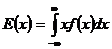# Mathematical Expectation And Generating Function Assignment Help

## 4. Mathematical Expectation And Generating Function

### 4.1 Mathematical Expectation:

The mathematical expression for computing the expected value of a discrete random variables X with probability mass function (p.m.f) P(X) is

E(x) = ∑xp(x) { for discrete random variable}The mathematical expression for computing the expected value of a continuous random variables X with probability density function (p.d.f) f(X) is## Mathematical Expectation And Generating Function Assignment Help Through Online Tutoring and Guided Sessions from AssignmentHelp.Net